Courses

# JEE Main Mathematics Mock - 8

## 25 Questions MCQ Test Mock Test Series for JEE Main & Advanced 2022 | JEE Main Mathematics Mock - 8

Description
This mock test of JEE Main Mathematics Mock - 8 for JEE helps you for every JEE entrance exam. This contains 25 Multiple Choice Questions for JEE JEE Main Mathematics Mock - 8 (mcq) to study with solutions a complete question bank. The solved questions answers in this JEE Main Mathematics Mock - 8 quiz give you a good mix of easy questions and tough questions. JEE students definitely take this JEE Main Mathematics Mock - 8 exercise for a better result in the exam. You can find other JEE Main Mathematics Mock - 8 extra questions, long questions & short questions for JEE on EduRev as well by searching above.
QUESTION: 1

Solution:
QUESTION: 2

Solution:
QUESTION: 3

### The area, in square unit, of the region bounded by the curve x2 = 4y , the line x = 2 and the x-axis is

Solution:
QUESTION: 4

The radical axis of the circles, belongs to the coaxial system of circles whose limiting points are (1,3) and (2,6) is

Solution:
QUESTION: 5

If y=sin((1+x2)/(1-x2)), (dy/dx)=

Solution: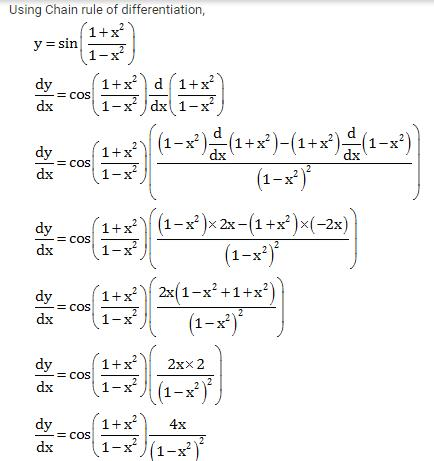QUESTION: 6

If z₁ and z₂ are any two complex numbers then |z₁ + z₂|2 + |z₁ - z₂|2 =

Solution:
QUESTION: 7

If the line 2x - y + k = 0 is a diameter of the circle x2 + y2 + 6x -6y + 5 =0, then k is equal to

Solution:
QUESTION: 8
The solution of the differential equation cos x sin y dx + sin x cos y dy =0 is
Solution:
QUESTION: 9

The complex equation |z + 1 − i| = |z + i − 1| represents a

Solution:
QUESTION: 10
The minimum value of sinx + cosx is
Solution:
QUESTION: 11

Which of the following is a Contradiction ?

Solution:
QUESTION: 12

In the following question, a Statement of Assertion (A) is given followed by a corresponding Reason (R) just below it. Read the Statements carefully and mark the correct answer-
Assertion (A): Three vectors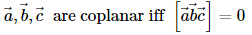Reason (R): The coplanar vectors are linearly independent

Solution:
QUESTION: 13

In the following question, a Statement-1 is given followed by a corresponding Statement-2 just below it. Read the statements carefully and mark the correct answer-
Consider the system of equations
x - 2y + 3z = -1
- x + y - 2z = k
x - 3y + 4z = 1
Statement-1:
The system of equations has no solution for k ≠3.
Statement-2:
The determinant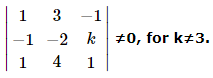Solution:
QUESTION: 14
If the traces of the matrices A and B are 20 and -8, then the trace of (A + B)=
Solution:
QUESTION: 15

Twelve students compete for a race. The number of ways in which first three prizes can be taken is

Solution:
QUESTION: 16

The tangents at the points a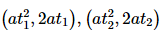on the parabola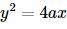are at right angles if

Solution:
QUESTION: 17
If the coefficient of correlation between x and y is 0.28, covariance between x and y is 7.6, and the variance of x is 9, then the standard deviation of the y series is
Solution:
QUESTION: 18

Points (0,0), (2,-1) and (9,2) are vertices of a triangle, then cosB =

Solution:
QUESTION: 19
8 coins are tossed simultaneously. The probability of getting at least 6 heads is
Solution:
QUESTION: 20

The angle between the lines (x - 1)/1 = (y - 1)/1 = (z - 1)/2 and (x - 1)/(- √3 - 1) = (y - 1)/(√3 - 1) = (z - 1)/4 is

Solution:
*Answer can only contain numeric values
QUESTION: 21

If function ƒ(x) = x3 + ax2 + bx + c is monotonically increasing ∀ x ∈ R, where a & b are prime numbers less than 10, then number of possible ordered pairs (a,b) is

Solution:

a, b ∈ {2, 3, 5, 7}
ƒ '(x) = 3x2 + 2ax + b ≥ 0    ∀ x ∈ R
D ≤ 0
4a2 – 12b ≤ 0
4(a2 – 3b) ≤ 0
a = 2    b = 2,3,5,7
a = 3    b = 3,5,7
a = 5    No solution
a = 7    No solution
Ordered pairs (a,b) are (2,2), (2,3), (2,5), (2,7), (3, 3), (3,5) & (3,7)

*Answer can only contain numeric values
QUESTION: 22

Suppose that ƒ is differentiable for all x such that ƒ'(x) ≤ 2 for all x. If ƒ(1) = 2 and ƒ(4) = 8 then ƒ(2) has the value equal to -

Solution: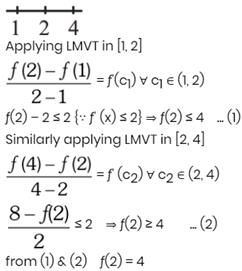*Answer can only contain numeric values
QUESTION: 23

Consider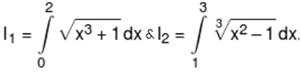then I1 + I2 is equal to

Solution: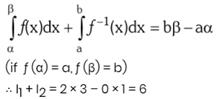*Answer can only contain numeric values
QUESTION: 24

If m =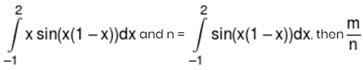is equal to:-

Solution: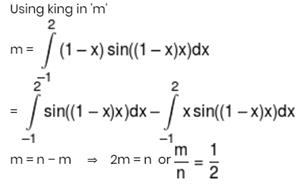*Answer can only contain numeric values
QUESTION: 25

Let y = g(x) be the inverse of a bijective mapping f : R → R, f(x) = 3x3 + 2x. The area bounded by graph of g(x), the x-axis and the ordinate at x = 5 is -

Solution: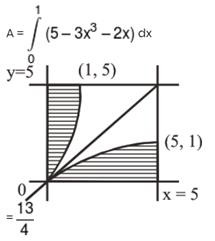Track your progress, build streaks, highlight & save important lessons and more!

### Similar Content### Related tests